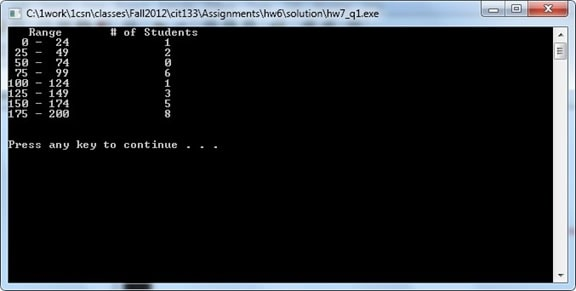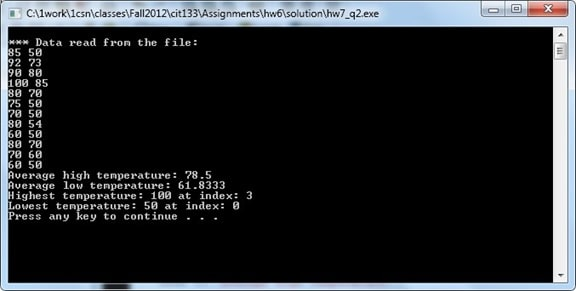# CIT133 Assignment 7 Chapter 8

1. Write the program for exercise 4 at the end of chapter 8. This is the program that reads a file consisting of students’ test scores. You can use the attached file named studentData.txt to test your code. I NEED functions. At this level, if your programs are not modular, I and you are in trouble! You need to have modularized programs. You MUST use arrays when needed. In addition to the requirements in the textbook, a) sort the data read from the file in ascending order
2. sort the data in descending order. Functions to sort the data will be required.
3. ask the user for a test score and display the location of the given value in the original list. So, if the user enters 150, the location will be 3, 9 and 16. Also, report the number of times this given data value was found in the list. Of course, if the given data value does not exist in the file, display an appropriate message.
4. ask the user for his or her full name (including spaces such as: joe smith). Store the data in a character array and output the number of characters in the name and convert it to all upper case letters. Use functions.

Here is the range output, if you use my data file:1. Write the program for exercise 9 at the end of chapter 8. This is the exercise that uses a two-dimensional array to store the highest and lowest temperatures for each month of the year.

You may want to create your tw-dimensional array as: int dataArray[NO_OF_MONTHS]; of course, NO_OF_MONTHS could be a constant equal to 12. Now, you have the following structure:

 85 50 92 73 90 80 Etc.

Note that the rows go from 0-11 and columns go from 0 to 1. In this example, the first month, has a high of 85 and low of 50. It’ll be a good idea to read the data from a file, so you don’t have to keep entering data. You may want to use my data file

(temperaturedata.txt). In addition to steps a through f, I also need the entire data read from the file displayed on the screen using a function. The data must be presented in rows and columns.

Here is some partial output from the given file: